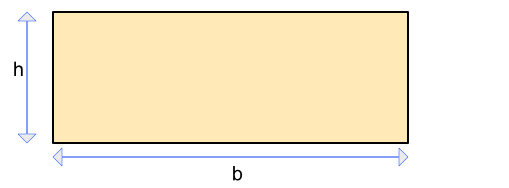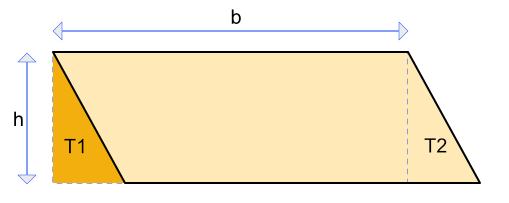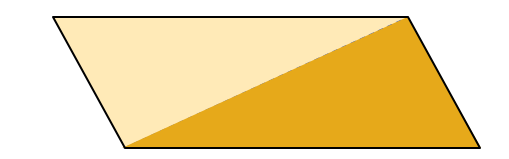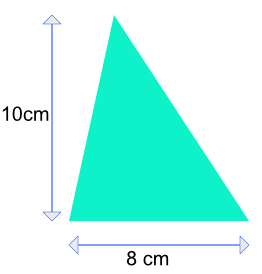Area of a Triangle

# Area of a Triangle

GCSE(F),

The area of a triangle is given by:

frac(1)(2)bh

where b is the distance along the base of the triangle, and h is the vertical height of the triangle.

This can be deduced from the area of a rectangle, which is given by A = bhMove a triangle from one end of the rectangle (T1), and position on the other end (at T2). The area of the parallelogram is the same as the area of the rectangle.The area of a triangle is half the area of the parallelogram.## Examples

1. What is the area of this triangle?Area of a triangle = frac(1)(2)bh

= frac(1)(2) x 10 x 8

= 40

2. A triangle is twice as high as it is wide. If the area of the triangle is 32 cm2, what is the height of the triangle?

Area of a triangle = frac(1)(2)bh
32 = x xx 2x, where x is the base
32 = 2x^2
16 = x^2
4 = x